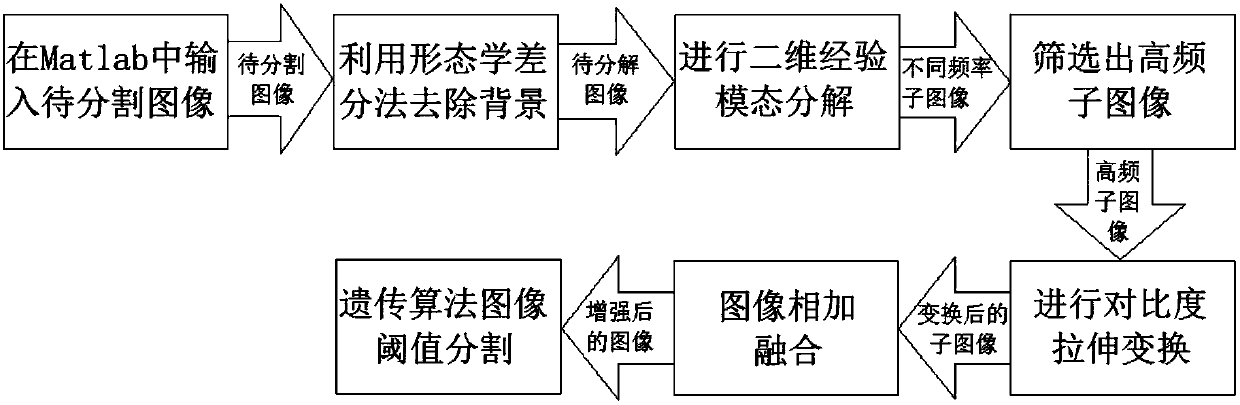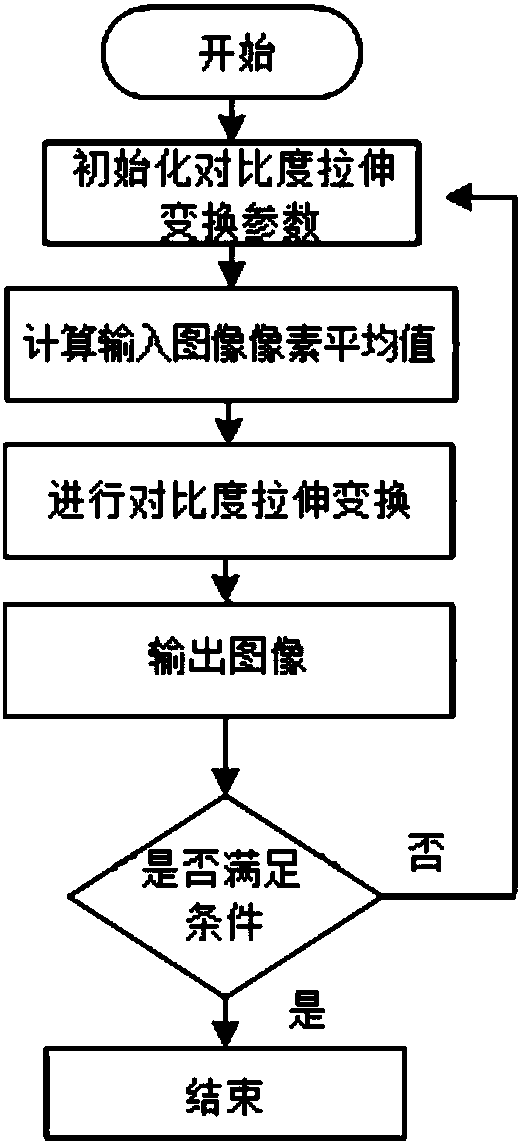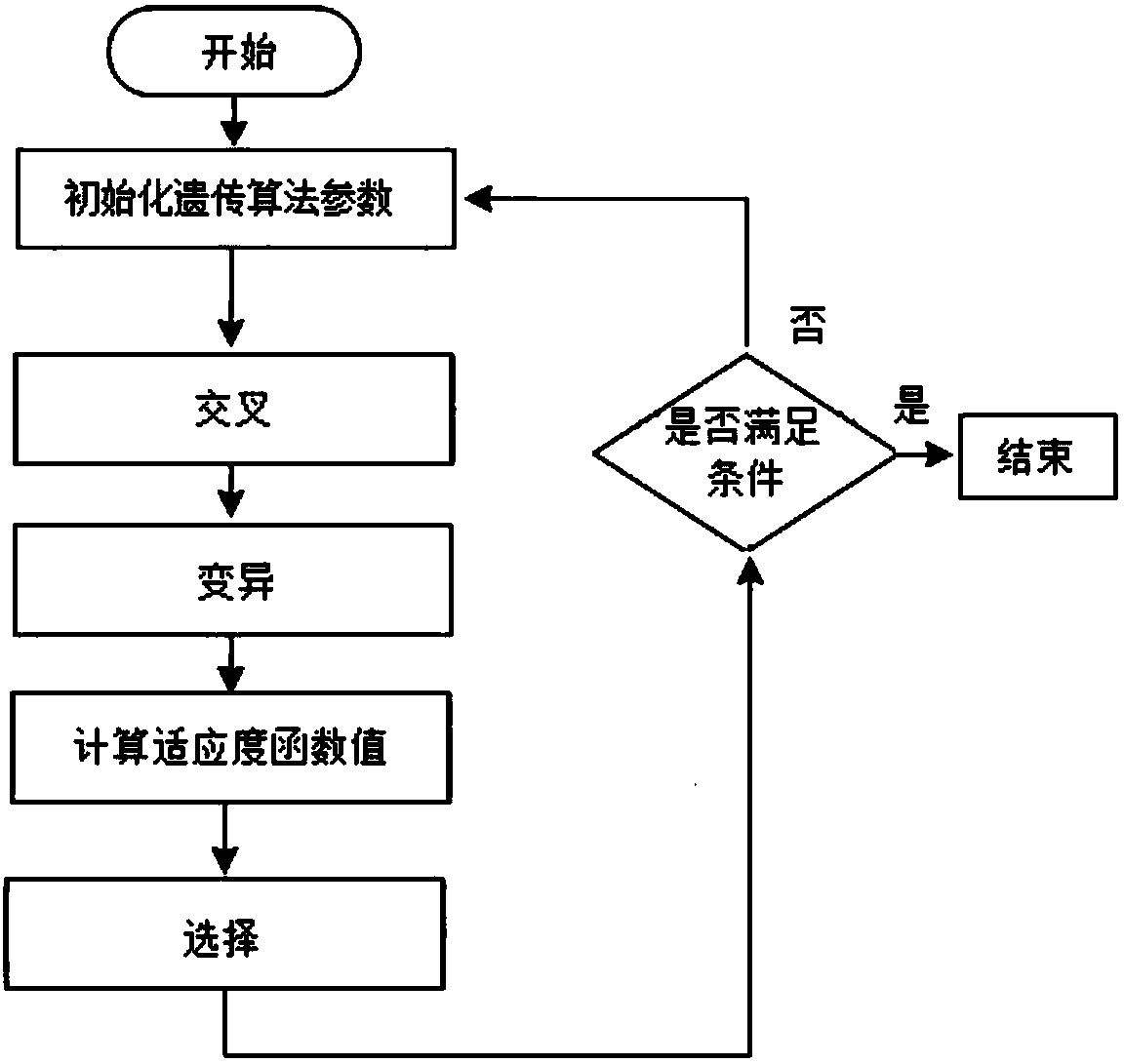# Image threshold segmentation method based on two-dimensional empirical mode decomposition and genetic algorithm

## A technology of empirical mode decomposition and genetic algorithm, which is applied in the field of image threshold segmentation, can solve problems such as not easy to determine the optimal segmentation threshold, over-segmentation, noise sensitivity, etc., and achieve good and complete segmentation results

Inactive Publication Date: 2018-08-28
KUNMING UNIV OF SCI & TECH
2 Cites 1 Cited by

## AI-Extracted Technical Summary

### Problems solved by technology

Threshold segmentation is a very simple image segmentation method, but it will encounter optimization problems when processing images based on this method, and it is not easy to determine the optimal segmentation threshold; region growth segmentation was first proposed by Levine et al. The image segmentation method of serial area segmentation, although its idea is relatively simple, it can usually segment connected areas with the same characteristics, and can provide good boundary information and segment...
View more

## Abstract

The invention relates to an image threshold segmentation method based on two-dimensional empirical mode decomposition and a genetic algorithm. A to-be-segmented image is inputted and the background ofthe image is removed by using a morphological difference method to obtain difference image; decomposition is carried out based on a two-dimensional empirical mode decomposition algorithm to obtain aseries of intrinsic mode functions and remainders; low-frequency sub-images that cannot express detailed information are removed and high-frequency sub-images are screened out; on the basis of a contrast stretching and transformation algorithm, contrast stretching and transformation are carried out on the high-frequency sub-images; the transformed high-frequency sub-images are added and fused to obtain an enhanced image; and threshold segmentation is carried out on the enhanced image by using a genetic algorithm. According to the invention, in terms of segmentation effect, the image thresholdsegmentation method has the improved target segmentation effect and good segmentation integrity by being compared with the way of carrying out genetic-algorithm-based threshold segmentation directly on the image without any distinguishing; and in terms of the feature similarity, the image threshold segmentation method obtains a value higher than that obtained by genetic-algorithm-based threshold segmentation directly on the image.

Application Domain

Image enhancementImage analysis +1

Technology Topic

Computer visionDecomposition +8

## Image

•••## Examples

• Experimental program(1)

### Example Embodiment

 Example 1: as Figure 1-12 As shown, an image threshold segmentation method based on two-dimensional empirical mode decomposition and genetic algorithm, input an image to be segmented, remove the background of the image by the morphological difference method, and obtain the difference image d(x, y), and then A series of intrinsic mode functions IMF(x, y) and residual REF are obtained by decomposing the two-dimensional empirical mode decomposition algorithm; the series of intrinsic mode functions IMF(x, y) are expressed as sub-images of different frequencies. The low-frequency sub-images showing detailed information, and the high-frequency sub-images are screened out; the contrast-stretching transformation algorithm is used to perform contrast-stretching transformation on the high-frequency sub-images; then the transformed high-frequency sub-images are added and fused to obtain an enhanced image. Image H; finally, the enhanced image is thresholded by genetic algorithm.
 Further, the image threshold segmentation method based on two-dimensional empirical mode decomposition and genetic algorithm can be set, specifically:
 Step 1. Input an image f(x,y) to be segmented in Matlab (such as Figure 4 The original Eyeball image shown), use the morphological difference method to remove the background image b(x,y), and obtain the difference image d(x,y):
 d(x,y)=f(x,y)-b(x,y)(1)
 Among them, x is the abscissa of the image, y is the ordinate of the image;
 Step 2. Perform two-dimensional empirical mode decomposition on the difference image d(x, y), and decompose it into z intrinsic mode functions IMF(x, y) and the remainder REF:

 Among them, REF is the residual function after the decomposition of the two-dimensional empirical mode, that is, the residual function of the last decomposition.
 The two-dimensional empirical mode decomposition of the difference image d(x,y) is specifically:
 Step 2.1. Take the difference image d(x, y) as the object of the two-dimensional empirical mode decomposition, assign the initial value m=1, n=1, and the remainder function r mn (x,y)=d(x,y), SD=1; where, the variable m represents the number of intrinsic mode functions IMF(x,y), and the variable n represents the number of IMFs used to calculate the IMF m (x, y) the nth cycle performed; SD represents the standard deviation;
 Step 2.2, calculate r mn (x,y) maximum value envelope w up (x,y) and its minimum envelope w low (x,y);
 Step 2.3, calculate r mn The mean surface of (x,y): mean(x,y)=(w up (x,y)+w low (x,y))/2;
 Step 2.4, according to r mn (x,y) and its mean surface mean(x,y) to calculate its difference function, and the difference calculation formula is as follows: D mn (x,y)=r mn (x,y)-mean(x,y);
 Step 2.5, take the iteration termination parameter ε=0.3, X and Y represent the maximum value of x and y, and the termination condition that satisfies the intrinsic mode function is:
 Step 2.6, judge the difference function D mn Whether (x, y) satisfies the termination condition of the intrinsic mode function described in step 2.5, namely SD
 When satisfied, the intrinsic mode function: IMF m (x,y)=D mn (x,y), r (m+1)n (x,y)=r mn (x,y)-IMF m (x, y), and let m=m+1, n remain unchanged, r mn (x,y)=r (m+1)n (x, y), repeat steps 2.2 to 2.6 to calculate the next intrinsic mode function, until the residual function has no extremum points and there will be no maximum points and minimum values ​​after the multi-order derivation of the residual function. point;
 When not satisfied, r m(n+1) (x,y)=D mn (x, y), and let n=n+1, the value of m remains unchanged, r mn (x,y)=r m(n+1) (x, y), repeat steps 2.2 to 2.6 until the difference function satisfies the termination condition of the intrinsic mode function;
 Step 2.7. Through steps 2.1-2.6, the difference image d(x,y) is decomposed into z intrinsic mode functions IMF m (x,y) and remainder REF: Among them, REF is the residual function after the decomposition of the two-dimensional empirical mode, that is, the residual function of the last decomposition.
 Step 3: Screen the original difference image d(x, y) through a series of intrinsic mode functions obtained after two-dimensional empirical mode decomposition, that is, a series of sub-image IMF(x, y), and retain high-frequency sub-image IMFs 1 (x,y),IMF 2 (x,y),...IMF b (x,y), discard low-frequency sub-image IMF b+1 (x,y),IMF b+2 (x,y),...,IMF z (x, y) and the remainder REF; where b is the total number of high-frequency sub-images. In this embodiment, b=2 and z=3.
 Step 4: Contrast stretching transformation is performed on the high-frequency sub-images respectively, so as to obtain the high-frequency sub-images after contrast-stretching transformation. The contrast-stretching transformation formula is as follows:
 s=T(j)=1/(1+(g/r) E )(3)
 Among them, r represents the gray level of the corresponding point (x, y) of the input image j, s is the output image, T is the contrast stretching transformation function, and the control contrast stretching transformation slope parameter E=0.9, the pixel average value of the input image g= sum/(X×Y), sum is the sum of the pixels of the input image, and X and Y represent the maximum values ​​of the variables x and y, respectively.
 Step 5. Add and fuse the high-frequency sub-images after contrast stretching to obtain the enhanced image H:
 H=T(IMF 1 (x,y))+T(IMF 2 (x,y))+...+T(IMF b (x,y))(4)
 Among them, H is the enhanced image after fusion, and T is the contrast stretching transformation function.
 Step 6. Randomly generate initial chromosomes encoded by real numbers in the image H, and form a chromosome group, that is, a population, and the number of chromosomes is 10; the population size is 10;
 Step 7. Perform crossover and mutation operations on the population first. The crossover probability is 0.7, and the mutation probability is 0.4. The parent chromosomes are randomly paired in pairs. For each parent p 1 ,p 2 Perform first crossover and then mutation to generate mutated offspring c 1 ,c 2 :
 For the crossover, the specific formula is:

 Among them, a 1 ,a 2 is the two offspring after the crossover, p 1 ,p 2 for two parents; is the expansion factor, μ is a random number in [0,1], η c =15 is represented as a cross index;
 The formula for the variation is:
 c k =a k +δ(U k max -U k min )(7)
 where c k represents the mutated offspring, a k is the offspring after crossover, U k max and U k min respectively a k The upper and lower limits of the value range of the variation point, k is 1, 2; the parameter δ is obtained through the polynomial probability distribution l is a random number in [0,1], η m =20 is expressed as mutation index.
 Step 8. Use the fitness function lownum×highnum×[(lowsum÷lownum)-(higsum÷highnum)] 2 Calculate the fitness value of the chromosome to evaluate the quality of the chromosome, where lownum is the total number of pixels below the chromosome size after crossover mutation, highnum is the total number of pixels higher than the chromosome size after crossover mutation, and lowsum is lower than the crossover mutation. The sum of the gray value of the pixels after the chromosome size, higsum is the sum of the gray values ​​of the pixels higher than the size of the chromosome after the cross mutation;
 Step 9. Calculate the mutated offspring c 1 ,c 2 fitness value of like i=1,2, then use c i replace p i , otherwise the probability keep p i;in, is the fitness value of the parent, f p is the sum of the fitness values ​​of all individuals in the population.
 Step 10. Repeat steps 7-9 for the new population until the maximum number of iterations is 150; the value of the chromosome with the highest fitness value obtained after 150 iterations is the optimal threshold
 Step 11. Use the optimal threshold obtained by the above-mentioned genetic algorithm to perform threshold segmentation, and the formula is as follows:

 Among them, f(q) is the pixel value of each point of the H image after threshold segmentation, q is the pixel value of each point of the H image, and v is the optimal threshold.
The accompanying drawings in the description show the results obtained by performing morphological difference processing on the original image according to the present invention; the results are as follows: Figure 5 shown, from Figure 5 It can be seen that the background information is basically removed.
 The accompanying drawings of the description also show the results obtained by performing two-dimensional empirical mode decomposition on the image after morphological difference processing in the present invention; the results are three IMF (x, y) component IMFs 1 (x,y),IMF 2 (x,y),IMF 3 (x,y) and a remainder function REF. from Image 6 It can be seen that the first component obtained after BEMD decomposition is IMF 1 (x,y) contains rich boundary and detail information; from Figure 7 The second component IMF obtained after BEMD decomposition can be seen in 2 (x,y), IMF 2 (x,y) than IMF 1 (x,y) is fuzzy, but still has rich boundary information; from Figure 8 The third component IMF obtained after BEMD decomposition can be seen in 3 (x,y), IMF 3 (x,y) contains more low-frequency components; from Figure 9 It can be seen that the remainder of the image obtained after BEMD decomposition is REF, and REF is relatively blurred and mainly consists of low-frequency components, representing image trend information. It can be seen from the results that as m increases, images of different frequencies can be decomposed.
 by MATLAB program Image 6 and Figure 7 Contrast stretching transformation is performed separately, and then additive fusion is performed.
 The result is as Figure 10 shown. As a result, it can be seen that the target information is enhanced and clear.
 Images that are directly segmented by genetic algorithm thresholding such as Figure 11 As shown, the segmentation result of the present invention is as follows Figure 12 shown, it can be seen that Figure 11 A large amount of information of interest is over-segmented and there is information loss, Figure 12 The target information is complete and clear, and the information of interest is almost all segmented.
 In order to express the effect of the present invention more intuitively and clearly, the following two evaluation methods are used to analyze the segmentation effect and image quality evaluation of the present invention.
 The method of the present invention records its optimal threshold, peak signal-to-noise ratio (PSNR) and feature similarity (FSIM) values ​​by running in Matlab; and compares the algorithm in this paper with the direct genetic algorithm threshold segmentation, as shown in Table 1 Show. The larger the peak signal-to-noise ratio, the better the image quality; the value range of the feature similarity is [0, 1], and the closer the value is to 1, the better the quality of the segmented image.
 Comprehensive analysis of the data in Table 1, wherein, PSNR represents peak signal-to-noise ratio, FSIM represents feature similarity, the PSNR value segmented by the method of the present invention is higher than the direct genetic algorithm threshold segmentation, and the FSIM segmented by the method of the present invention is also higher than the direct genetic algorithm threshold value segmentation. The segmentation result of the method of the present invention is better than that of the direct genetic algorithm threshold segmentation, and it can be seen that the optimal segmentation threshold of the direct genetic algorithm threshold segmentation is 117, and the optimal segmentation threshold of the present invention is 48.
 Table 1. Image segmentation effect evaluation based on FSIM and SSIM
 method

## PUM## Description & Claims & Application Information

We can also present the details of the Description, Claims and Application information to help users get a comprehensive understanding of the technical details of the patent, such as background art, summary of invention, brief description of drawings, description of embodiments, and other original content. On the other hand, users can also determine the specific scope of protection of the technology through the list of claims; as well as understand the changes in the life cycle of the technology with the presentation of the patent timeline. Login to view more.
Who we serve
• R&D Engineer
• R&D Manager
• IP Professional
Why Eureka